Time: 3 Hours                                           DECEMBER 2006                                                        Max. Marks: 100

NOTE: There are 9 Questions in all.

·      Question 1 is compulsory and carries 20 marks. Answer to Q. 1. must be written in the space provided for it in the answer book supplied and nowhere else.

·      Out of the remaining EIGHT Questions answer any FIVE Questions. Each question carries 16 marks.

·      Any required data not explicitly given, may be suitably assumed and stated.

Q.1       Choose the correct or best alternative in the following:                                         (2x10)

a.       The value of limit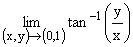(A)  0                                                  (B)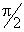(C)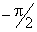(D)  does not exist

b.      Let a function f(x, y) be continuous and possess first and second order partial derivatives at a point (a, b).  If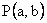is a critical point and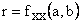,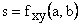,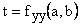then the point P is a point of relative maximum if

(A)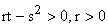(B)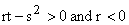(C)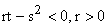(D)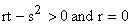c.   The triple integral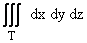gives

(A)    volume of region T                        (B)  surface area of region T

(C)  area of region T                            (D)  density of region T

d.   If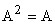then matrix A is called

(A)    Idempotent Matrix                      (B) Null Matrix

(C)  Transpose Matrix                        (D)  Identity Matrix

e.   Let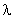be an eigenvalue of matrix A then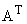, the transpose of A, has an eigenvalue as

(A)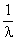(B)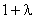(C)(D)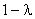f.    The system of equations is said to be inconsistent, if it has

(A)     unique solution                             (B)  infinitely many solutions

(C)  no solution                                    (D) identity solution

g.   The differential equation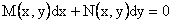is an exact differential equation if

(A)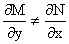(B)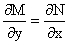(C)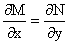(D)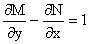h.   The integrating factor of the differential equation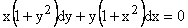is

(A)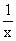(B)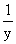(C) xy                                                 (D)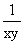i.    The functions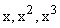defined on an interval I, are always

(A)   linearly dependent                         (B) homogeneous

(C) identically zero or one                    (D) linearly independent

j.    The value of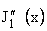, the second derivative of Bessel function in terms of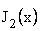and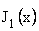is

(A)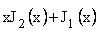(B)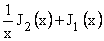(C)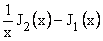(D)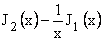Answer any FIVE Questions out of EIGHT Questions.

Each question carries 16 marks.

Q.2     a.   Show that the function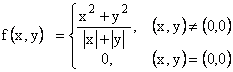is continuous at (0, 0) but its partial derivatives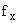and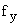do not exist at (0, 0).                (8)

b.   Find the linear and the quadratic Taylor series polynomial approximation to the function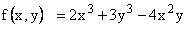about the point (1, 2).  Obtain the maximum absolute error in the region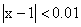and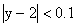for the two approximations.                                                (8)

Q.3     a.   Find the shortest distance between the line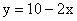and the ellipse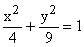.                   (8)

b.   Evaluate the double integral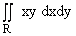, where R is the region bounded by the x-axis, the line y = 2x and the parabola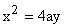.                      (8)

Q.4     a.   Evaluate the integral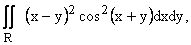where R is the parallelogram with successive vertices at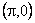,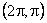,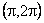and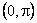.      (8)

b.   Show that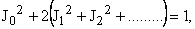where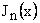is the Bessel function of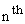order.                                                                     (8)

Q.5     a.   Show that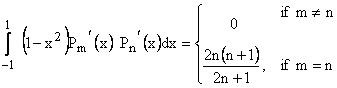.                    (6)

where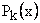are the Legendre polynomials.

b.   Find the power series solution about x =2, of the initial value problem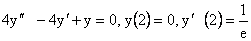.

Express the solution in closed form.                                                                  (10)

Q.6     a.   Solve the initial value problem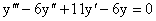y(0) = 0,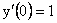,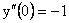.              (8)

b.   Solve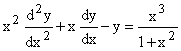.                                                                 (8)

Q.7     a.   Show that set of functions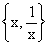forms a basis of the differential equation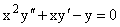.  Obtain a particular solution when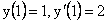.                                                         (6)

b.   Solve the following differential equations:

(i)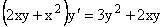(ii)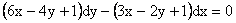(2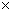5 = 10)

Q.8     a.   Let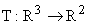be a linear transformation defined by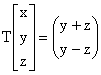.  Taking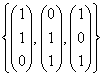as a basis in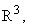determine the matrix of linear transformation.                                                  (8)

b.   If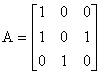then show that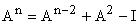, for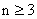.     Hence find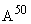.             (8)

Q.9     a.   Examine whether matrix A is similar to matrix B, where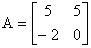,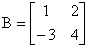.                 (8)

b.   Discuss the consistency of the following system of equations for various values of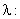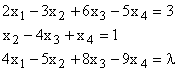and if consistent, solve it.                                                                                    (8)